Analog Electronics - 4

# Analog Electronics - 4

Test Description

## 10 Questions MCQ Test GATE ECE (Electronics) 2023 Mock Test Series | Analog Electronics - 4

Analog Electronics - 4 for Electronics and Communication Engineering (ECE) 2023 is part of GATE ECE (Electronics) 2023 Mock Test Series preparation. The Analog Electronics - 4 questions and answers have been prepared according to the Electronics and Communication Engineering (ECE) exam syllabus.The Analog Electronics - 4 MCQs are made for Electronics and Communication Engineering (ECE) 2023 Exam. Find important definitions, questions, notes, meanings, examples, exercises, MCQs and online tests for Analog Electronics - 4 below.
Solutions of Analog Electronics - 4 questions in English are available as part of our GATE ECE (Electronics) 2023 Mock Test Series for Electronics and Communication Engineering (ECE) & Analog Electronics - 4 solutions in Hindi for GATE ECE (Electronics) 2023 Mock Test Series course. Download more important topics, notes, lectures and mock test series for Electronics and Communication Engineering (ECE) Exam by signing up for free. Attempt Analog Electronics - 4 | 10 questions in 30 minutes | Mock test for Electronics and Communication Engineering (ECE) preparation | Free important questions MCQ to study GATE ECE (Electronics) 2023 Mock Test Series for Electronics and Communication Engineering (ECE) Exam | Download free PDF with solutions
 1 Crore+ students have signed up on EduRev. Have you?
Analog Electronics - 4 - Question 1

### The feedback topology of the given circuit is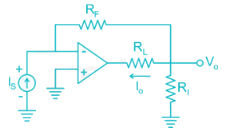Detailed Solution for Analog Electronics - 4 - Question 1

The input is current, current is added in parallel (Hence shunt).

From the output, voltage is sampled (shunt)

Hence shunt series feedback topology.

*Answer can only contain numeric values
Analog Electronics - 4 - Question 2

### In the circuit shown below all the transistors are identical with; VBE = 0.7 V; VCE(sat) = 0.2 If the transistor Q3 is in saturation then V0 is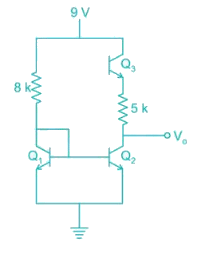Detailed Solution for Analog Electronics - 4 - Question 2

The collector currents of both Q1 and Q2 will be identical due to same base current.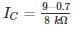Since Q3 is in saturation

9 – 0.2 – 5 × 103 IC - VO = 0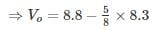Vo = 3.6 V

Analog Electronics - 4 - Question 3

### Consider series-shunt amplifier in which the open loop gain is Av = 105 and the closed loop gain is AVf = 50. Assume the input and output resistance of the basic amplifier are Ri = 10 kΩ and Ro = 20 kΩ. Determine the input resistance of series input connecting and the output resistance of a shunt output connection for an ideal feedback voltage amplifier.

Detailed Solution for Analog Electronics - 4 - Question 3

The ideal closed-loop voltage transfer function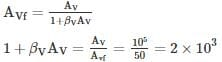The input resistance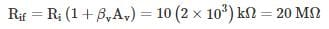The output resistance is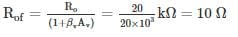Analog Electronics - 4 - Question 4

For the circuit shown in fig, the transconductance of Q1 is gm1, of Q2 is gm2 and Q3 is gm3 then the overall trans-conductance gm is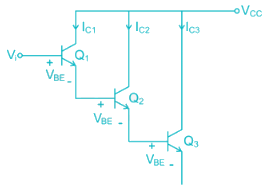Detailed Solution for Analog Electronics - 4 - Question 4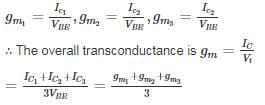*Answer can only contain numeric values
Analog Electronics - 4 - Question 5

The circuit shown in figure uses matched transistor. With a thermal voltage VT = 25 mV, The value of β is very high. Find the value of emitter resistance in kΩ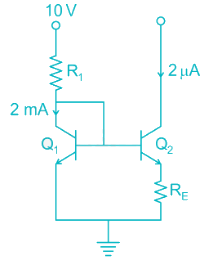Detailed Solution for Analog Electronics - 4 - Question 5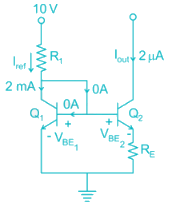Given circuit is widlar current source which is particularly suitable for low value of current.

Here Iout ≠ Iref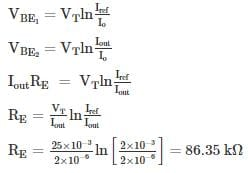Analog Electronics - 4 - Question 6

The two stages of the amplifier are shown in the figure. Both transistors have current gain β of 80 and dynamic emitter resistance r′e of 25Ω. The magnitude of the overall voltage gain of the amplifier is approximately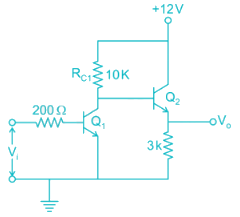Detailed Solution for Analog Electronics - 4 - Question 6

Objective approach

The second stage is emitter follower which has voltage gain of unity.

The overall voltage gain = gain of CE stage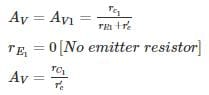rC1 = effective impedance seen by collector of transistor Q1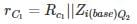Zi(base)Q2 at the base of transistor Q2.
The impedance Zi (base) Q2 at the base of transistor Q is
Zi(base)2 = βRE2
= 80 × 3 k

Zi (base) 2 = 240 kΩ

Therefore: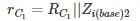= 10 k||240 k

rC1 = 9.6kΩ

Overall voltage gain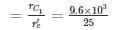AV− = 3 84

Subjective approach:

Draw the equivalent h-parameter model

rπ = β re = 80 x 25 = 2k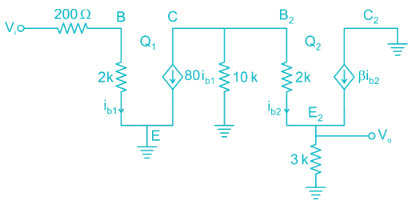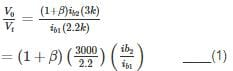Apply KVL in the base-emitter loop of transistor Q2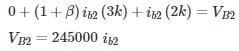Apply KCL at node VB2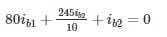800 ib1 = -255  ib2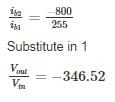*Answer can only contain numeric values
Analog Electronics - 4 - Question 7

Two RC coupled amplifiers are connected to form a 2-stage amplifier. If the lower and upper cut off frequencies of each individual amplifiers is 100 Hz and 20 kHz. Then the 3-dB bandwidth of the two-state amplifier is ____ Hz.

Detailed Solution for Analog Electronics - 4 - Question 7

Concept:

For multistage amplifier consisting of n identical amplifying states, the lower cut off fL (multi) is expressed as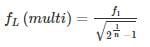Where f1 = lower cut off frequency of individual state.

Upper cut off frequency is given by: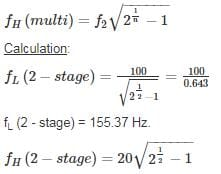= 20 × 0.643

= 12.87 kHz

Bandwidth =

= 12714.6 Hz.

Analog Electronics - 4 - Question 8

In the AC equivalent circuit shown in Fig, if Iin is the Input current and Feedback Resistance is very large, the type of Feedback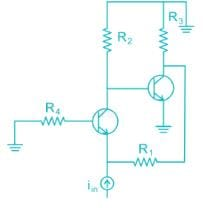Detailed Solution for Analog Electronics - 4 - Question 8

Procedure to identify the type of Feedback:

(1) Identify the element responsible for the Feedback.

(2) If Feedback element is directly connected to the output node, it indicates voltage sampling otherwise it indicates current sampling.

(3) If Feedback element is directly connected to the Input node it indicates shunt Mixing otherwise it indicate series mixing.

∴ It is voltage current Feedback.

*Answer can only contain numeric values
Analog Electronics - 4 - Question 9

The differential DC output voltage V01 – V02 in the circuit shown is ____ V.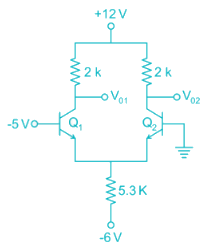Detailed Solution for Analog Electronics - 4 - Question 9

The base of Q1 is biased with negative voltage hence

Q1 → OFF

Q2 → ON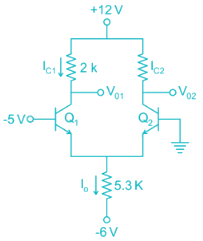IC1 = 0

V01 = 12 V

VE2 = -0.7 V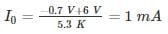V02 = 12 – (1 mA) (2k)

= 12 – 2

= 10 V

V01 – V02 = 12 – 10 = 2 V

Analog Electronics - 4 - Question 10

Find the value of VCE in the given figure.
Assume the base currents to be the same.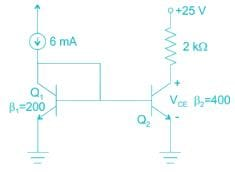Detailed Solution for Analog Electronics - 4 - Question 10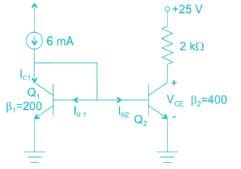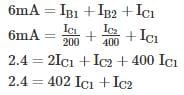Also we know IC = βIB

Since base currents are the same, we have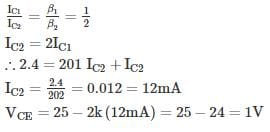## GATE ECE (Electronics) 2023 Mock Test Series

21 docs|263 tests
Information about Analog Electronics - 4 Page
In this test you can find the Exam questions for Analog Electronics - 4 solved & explained in the simplest way possible. Besides giving Questions and answers for Analog Electronics - 4, EduRev gives you an ample number of Online tests for practice

## GATE ECE (Electronics) 2023 Mock Test Series

21 docs|263 tests(Scan QR code)